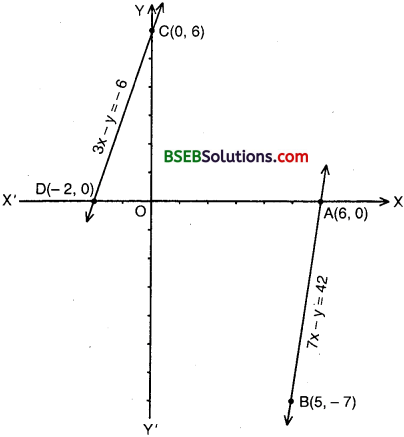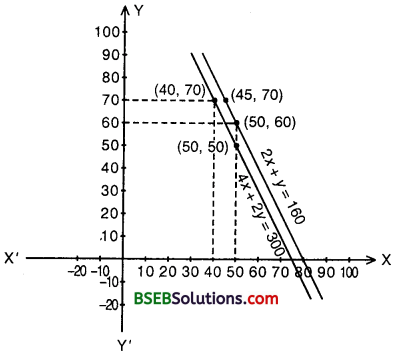# HSSlive: Plus One & Plus Two Notes & Solutions for Kerala State Board

## BSEB Class 10 Maths Chapter 3 Pair of Linear Equations in Two Variables Ex 3.1 Textbook Solutions PDF: Download Bihar Board STD 10th Maths Chapter 3 Pair of Linear Equations in Two Variables Ex 3.1 Book AnswersBSEB Class 10 Maths Chapter 3 Pair of Linear Equations in Two Variables Ex 3.1 Textbook Solutions PDF: Download Bihar Board STD 10th Maths Chapter 3 Pair of Linear Equations in Two Variables Ex 3.1 Book Answers

BSEB Class 10th Maths Chapter 3 Pair of Linear Equations in Two Variables Ex 3.1 Textbooks Solutions and answers for students are now available in pdf format. Bihar Board Class 10th Maths Chapter 3 Pair of Linear Equations in Two Variables Ex 3.1 Book answers and solutions are one of the most important study materials for any student. The Bihar Board Class 10th Maths Chapter 3 Pair of Linear Equations in Two Variables Ex 3.1 books are published by the Bihar Board Publishers. These Bihar Board Class 10th Maths Chapter 3 Pair of Linear Equations in Two Variables Ex 3.1 textbooks are prepared by a group of expert faculty members. Students can download these BSEB STD 10th Maths Chapter 3 Pair of Linear Equations in Two Variables Ex 3.1 book solutions pdf online from this page.

## Bihar Board Class 10th Maths Chapter 3 Pair of Linear Equations in Two Variables Ex 3.1 Books Solutions

 Board BSEB Materials Textbook Solutions/Guide Format DOC/PDF Class 10th Subject Maths Chapter 3 Pair of Linear Equations in Two Variables Ex 3.1 Chapters All Provider Hsslive

## How to download Bihar Board Class 10th Maths Chapter 3 Pair of Linear Equations in Two Variables Ex 3.1 Textbook Solutions Answers PDF Online?

2. Click on the Bihar Board Class 10th Maths Chapter 3 Pair of Linear Equations in Two Variables Ex 3.1 Answers.
3. Look for your Bihar Board STD 10th Maths Chapter 3 Pair of Linear Equations in Two Variables Ex 3.1 Textbooks PDF.
4. Now download or read the Bihar Board Class 10th Maths Chapter 3 Pair of Linear Equations in Two Variables Ex 3.1 Textbook Solutions for PDF Free.

## BSEB Class 10th Maths Chapter 3 Pair of Linear Equations in Two Variables Ex 3.1 Textbooks Solutions with Answer PDF Download

Find below the list of all BSEB Class 10th Maths Chapter 3 Pair of Linear Equations in Two Variables Ex 3.1 Textbook Solutions for PDF’s for you to download and prepare for the upcoming exams:

## BSEB Bihar Board Class 10th Maths Solutions Chapter 3 Pair of Linear Equations in Two Variables Ex 3.1

Question 1.
Aftab tells his daughter, “Seven years ago, I was seven times as old as you were then. Also, three years from now, I shall be three times as old as you will be”. (Isn’t this interesting)? Represent this situation algebraically and graphically.
Solution:
Let us denote the present age of daughter and her father Aftab as x years and y years respectively. Then, algebraic representation is given by the following equations:
7(x – 7) = y – 7 or 7x – 49 = y – 7
or 7x – y = 42 ………………. (1)
and 3(x + 3) = y + 3 or 3x + 9 = y + 3
or 3x – y = – 6 ……………….. (2)
To obtain the equivalent graphical representation, we find two points on the line representing each equation. That is, we find two solutions of each equation. These solutions are given below in the tables:
For 7x – y = 42For 3x – y = – 6To represent these equations graphically, we plot the points A(6, 0) and B(5, – 7) to get the graph of (1) and the points C(0, 6) and D(- 2, 0) give the graph of (2).Question 2.
The coach of a cricket team buys 3 bats and 6 balls for Rs 3900. Later, she buys another bat and 2 more balls of the same kind for Rs 1300. Represent this situation algebraically and geometrically.
Solution:
Let us denote the cost of one bat by Rs x and one ball by Rs y. Then, the algebraic representation is given by the following equations:
3x + 6y = 3900 ……………… (1)
and x + 2y = 1300 …………….. (2)
To obtain the equivalent geometric representation, we find two points on the line representing each equation. That is, we find two solutions of each equation. These solutions are given below in the table:We plot the points A(0, 650) and B(1300, 0) to obtain the geometric representation of 3x + 6y = 3900 and C(500, 400) and D(100, 600) to obtain the geometric representation of x + 2y = 1300.We observe that these lines are coincident.

Question 3.
The cost of 2 kg of apples and 1 kg of grapes on a day was found to be Rs 160. After a month, the cost of 4 kg of apples and 2 kg of grapes is Rs 300. Represent the situation algebraically and geometrically.
Solution:
Let us denote the cost of 1 kg of apples by Rs x and cost of 1 kg of grapes by Rs y.
Then the algebraic representation is given by the following equations:
2x + y = 160 …………….. (1)
4x + 2y = 300 ……………….. (2)
To find the equivalent geometric representation, we find two points on the line representing each equation. That is, we find two solutions of each equation.Geometric representation is shown on the previous pages which is a pair of parallel lines.

## Bihar Board Class 10th Maths Chapter 3 Pair of Linear Equations in Two Variables Ex 3.1 Textbooks for Exam Preparations

Bihar Board Class 10th Maths Chapter 3 Pair of Linear Equations in Two Variables Ex 3.1 Textbook Solutions can be of great help in your Bihar Board Class 10th Maths Chapter 3 Pair of Linear Equations in Two Variables Ex 3.1 exam preparation. The BSEB STD 10th Maths Chapter 3 Pair of Linear Equations in Two Variables Ex 3.1 Textbooks study material, used with the English medium textbooks, can help you complete the entire Class 10th Maths Chapter 3 Pair of Linear Equations in Two Variables Ex 3.1 Books State Board syllabus with maximum efficiency.

## FAQs Regarding Bihar Board Class 10th Maths Chapter 3 Pair of Linear Equations in Two Variables Ex 3.1 Textbook Solutions

#### How to get BSEB Class 10th Maths Chapter 3 Pair of Linear Equations in Two Variables Ex 3.1 Textbook Answers??

Students can download the Bihar Board Class 10 Maths Chapter 3 Pair of Linear Equations in Two Variables Ex 3.1 Answers PDF from the links provided above.

#### Can we get a Bihar Board Book PDF for all Classes?

Yes you can get Bihar Board Text Book PDF for all classes using the links provided in the above article.

## Important Terms

Bihar Board Class 10th Maths Chapter 3 Pair of Linear Equations in Two Variables Ex 3.1, BSEB Class 10th Maths Chapter 3 Pair of Linear Equations in Two Variables Ex 3.1 Textbooks, Bihar Board Class 10th Maths Chapter 3 Pair of Linear Equations in Two Variables Ex 3.1, Bihar Board Class 10th Maths Chapter 3 Pair of Linear Equations in Two Variables Ex 3.1 Textbook solutions, BSEB Class 10th Maths Chapter 3 Pair of Linear Equations in Two Variables Ex 3.1 Textbooks Solutions, Bihar Board STD 10th Maths Chapter 3 Pair of Linear Equations in Two Variables Ex 3.1, BSEB STD 10th Maths Chapter 3 Pair of Linear Equations in Two Variables Ex 3.1 Textbooks, Bihar Board STD 10th Maths Chapter 3 Pair of Linear Equations in Two Variables Ex 3.1, Bihar Board STD 10th Maths Chapter 3 Pair of Linear Equations in Two Variables Ex 3.1 Textbook solutions, BSEB STD 10th Maths Chapter 3 Pair of Linear Equations in Two Variables Ex 3.1 Textbooks Solutions,
Share: